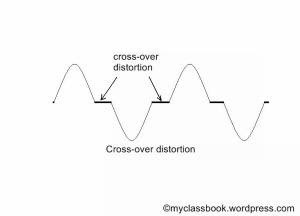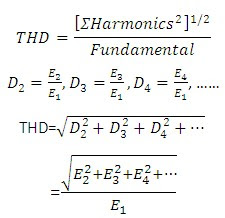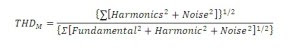# Harmonic distortion analyzers

## Harmonic distortion analyzers:

### Harmonic distortion:

When we give a sinusoidal signal input to any electronic instrument there should be output in sinusoidal form, but generally the output is not exactly the replica of input, because of various types of distortion that my occur.

Distortion is occur due to inherent non-linear characteristics of different components used in electronic circuit. Nonlinear behavior of electronic component introduces harmonics in the output waveform and the resultant distortion is often referred as harmonic distortion.

### 1)    Frequency distortion:

This type of distortion occurs in amplifiers because of amplification factor of amplifier is different for different frequencies.

### 2)    Amplitude distortion:

It occurs because amplifier introduces harmonic of fundamental of input frequency. Harmonics always generates distortion in amplitude. E.g. when amplifiers are overdriven it clips the waveform.

### 3)    Phase distortion:

This distortion occurs due to energy storage elements in the system which causes the output signal to be displaced in phase with the input signal. Signals with different frequencies will be shifted by different phase angles.

### 4)    Intermodulation distortion:

This type of distortion occurs as a consequence of interaction or heterodyning of two frequencies, giving an output which is sum or difference of the two original frequencies.

### 5)    Cross-over distortion:

This type of distortion occurs in push-pull amplifiers on account of incorrect boas levels as shown in figure.cross over distortion

### Total harmonic distortion (THD):In a measurement system noise is read in addition to harmonics and the total waveform consisting of harmonics, noise and fundamental is measured instead of fundamental alone